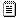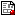[ Data Screening || Matrix Algebra with SAS/IML || Regression analysis || General Linear Models || Canonical correlation, Discriminant analysis || Logistic Regression || Factor Analysis || Clustering and scaling || SAS macro programs || Data sets || Programs]

## Matrix Algebra with SAS/IML

These tutorial examples use SAS/IML to illustrate various properties of matrix algebra and matrix operations.
imlasn4.sasGreen & Carroll problems
imldet1.sasProperties of determinants
imldet2.sasEvaluation of determinants by cofactor expansion and Gaussian elimination.
imleig.sasEigenvalues and Eigenvectors; properties of eigenvalues; illustrates the Spectral Decomposition of a matrix.
imleqn.sasSolution of Systems of Equations. Illustrates consistent & inconsistent equations, and the relation to matrix rank.
imlinv1.sasInverse of a matrix: definitions and properties.
imlinv2.sasMatrix inversion by elementary row operations
imlinv3.sasGenerailzed inverse of a matrix
imlmat1.sasBasic matrix definitions
imlmat2.sasMatrix arithmetic: +, -, # (elementwise operations)
imlmat4.sasProperties of matrix multiplication
imlproj.sasProjections: Projecting a vector in a vector space, and the Gram-Schmidt process.
imlrank.sasMatrix rank and linear independence
imlregr.sasRegression using the SWEEP operator in IML
imlreg1.sasRegression with IML
imlsscp.sasComputing Cross product Summary Matrices
imlxmp1.sasBasic matrix operations in Proc IML
matlib.sasSome useful IML modules for matrix operations